# Chapter 6 Aggregate demand aggregate supply Mentor Pham

• Slides: 51Chapter 6 Aggregate demand aggregate supply Mentor Pham Xuan Truong [email protected] edu. vnContent I Fluctuation of the economy in the short run and its trend in the long run II Aggregate demand Aggregate supply model (AD – AS model) III Explain behaviors of the economy via AD – AS model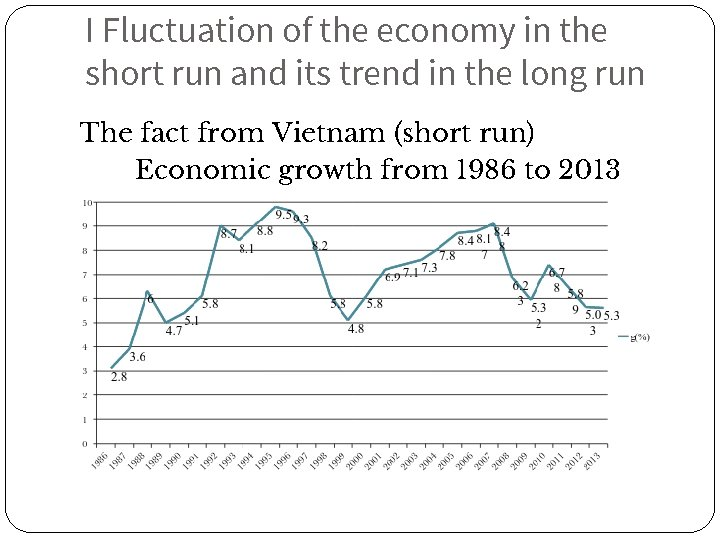I Fluctuation of the economy in the short run and its trend in the long run The fact from Vietnam (short run) Economic growth from 1986 to 2013I Fluctuation of the economy in the short run and its trend in the long run The fact from the US (long run) Economic growth from 1965 to 2010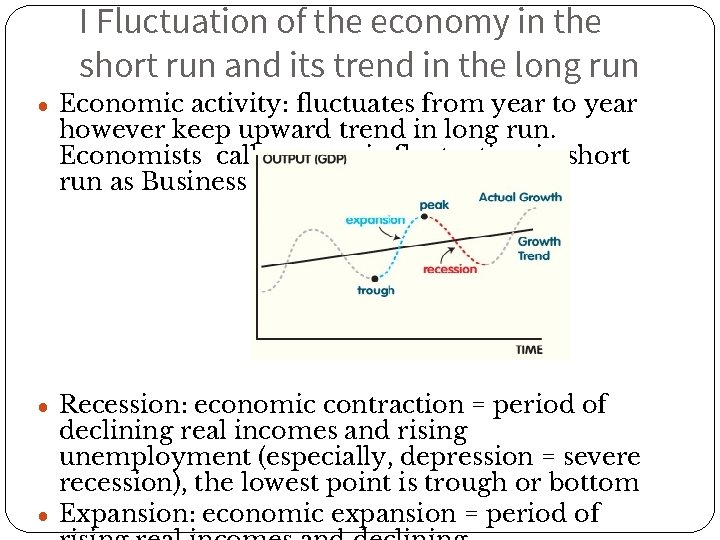I Fluctuation of the economy in the short run and its trend in the long run ● Economic activity: fluctuates from year to year however keep upward trend in long run. Economists call economic fluctuation in short run as Business cycle ● Recession: economic contraction = period of declining real incomes and rising unemployment (especially, depression = severe recession), the lowest point is trough or bottom ● Expansion: economic expansion = period ofI Fluctuation of the economy in the short run and its trend in the long run 3 key facts about economic fluctuations 1. Economic fluctuations are irregular and unpredictable 2. Most macroeconomic quantities fluctuate together 3. As output falls, unemployment rises This figure at the next slides will show real GDP in panel (a), investment spending in panel (b), and unemployment in panel (c) for the U. S. economy using quarterly data since 1965. Recessions are shown as the shaded areas.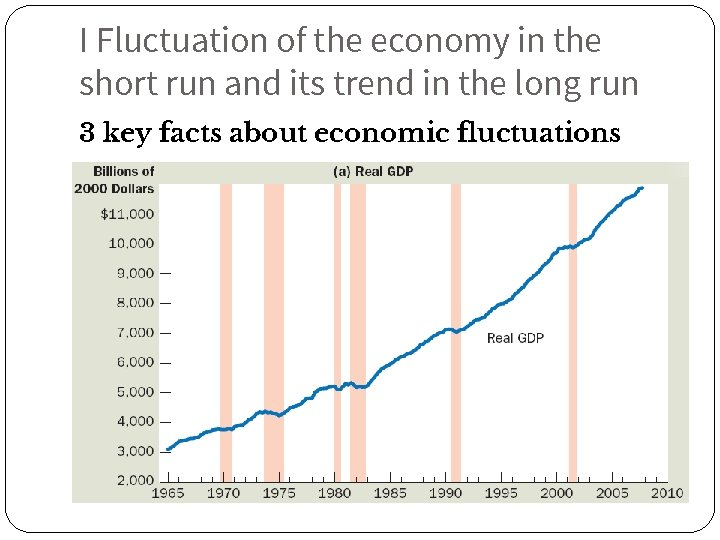I Fluctuation of the economy in the short run and its trend in the long run 3 key facts about economic fluctuations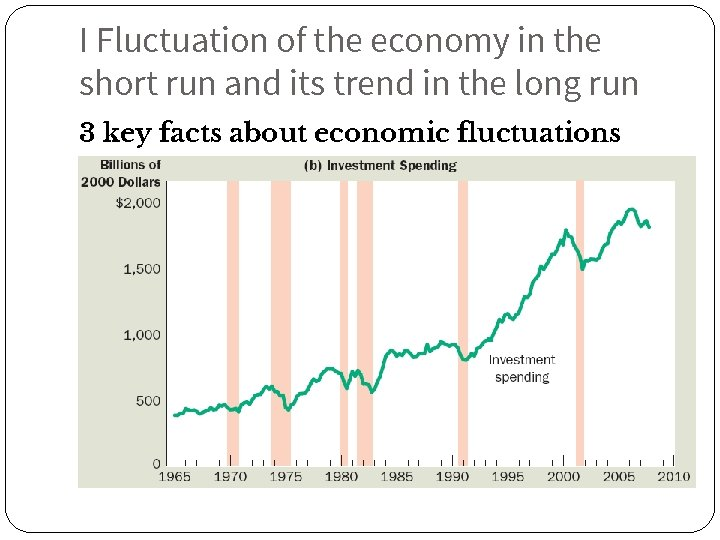I Fluctuation of the economy in the short run and its trend in the long run 3 key facts about economic fluctuations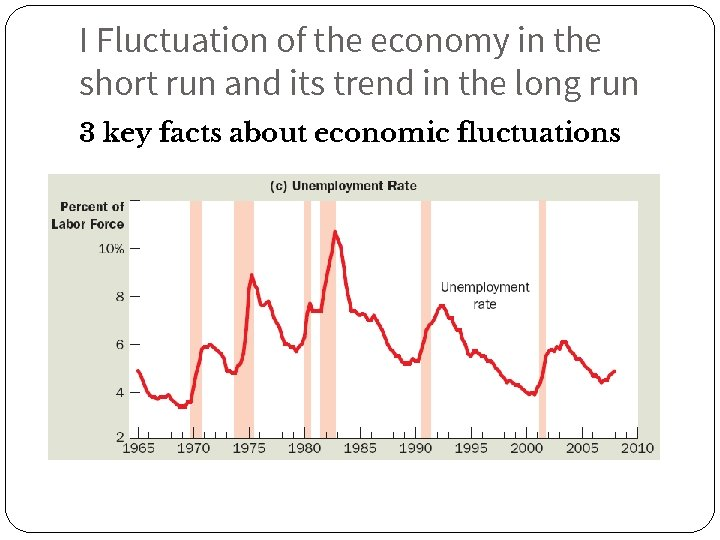I Fluctuation of the economy in the short run and its trend in the long run 3 key facts about economic fluctuations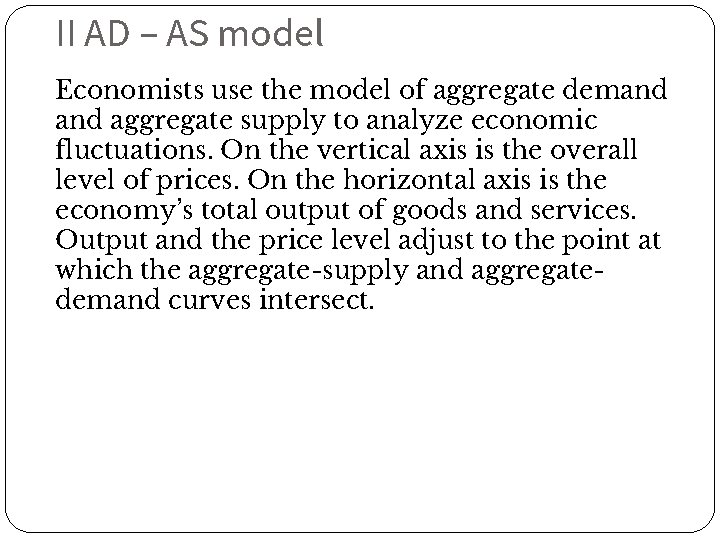II AD – AS model Economists use the model of aggregate demand aggregate supply to analyze economic fluctuations. On the vertical axis is the overall level of prices. On the horizontal axis is the economy’s total output of goods and services. Output and the price level adjust to the point at which the aggregate-supply and aggregatedemand curves intersect.II AD – AS model 1 Aggregate demand - Aggregate-demand curve shows the quantity of goods and services that households, firms, the government, and customers abroad want to buy at each price level - Aggregate demand curve is downward sloping In the next slides, we will examine two topics + Why the AD curve slopes downward + Why the AD curve might shiftII AD – AS model 1 Aggregate demand Why the aggregate-demand (AD) curve slopes downward Formula of AD: AD = C + I + G + NX ● Three effects that causes downward sloping AD curve ● Wealth effect (C ) ● Interest-rate effect (I) ● Exchange-rate effect (NX) ● Assumption: government spending (G) is fixed by policy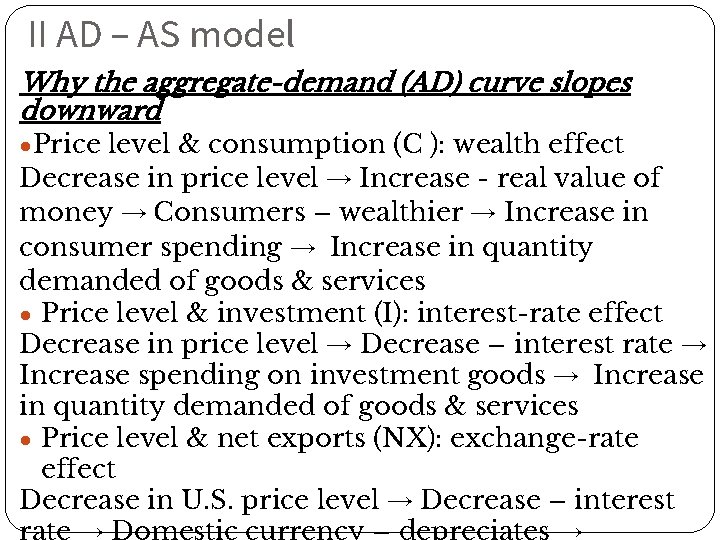II AD – AS model Why the aggregate-demand (AD) curve slopes downward ●Price level & consumption (C ): wealth effect Decrease in price level → Increase - real value of money → Consumers – wealthier → Increase in consumer spending → Increase in quantity demanded of goods & services ● Price level & investment (I): interest-rate effect Decrease in price level → Decrease – interest rate → Increase spending on investment goods → Increase in quantity demanded of goods & services ● Price level & net exports (NX): exchange-rate effect Decrease in U. S. price level → Decrease – interestII AD – AS model Why the aggregate-demand (AD) curve slopes downward ● A fall in price level increases quantity of goods& services demanded because: 1. Consumers are wealthier - stimulates the demand for consumption goods 2. Interest rates fall - stimulates the demand for investment goods 3. Currency depreciates - stimulates the demand for net exports ● A rise in price level Decreases quantity of goods and services demanded, because: 1. Consumers are poorer – depress consumer spending 2. Higher interest rates fall - depress investment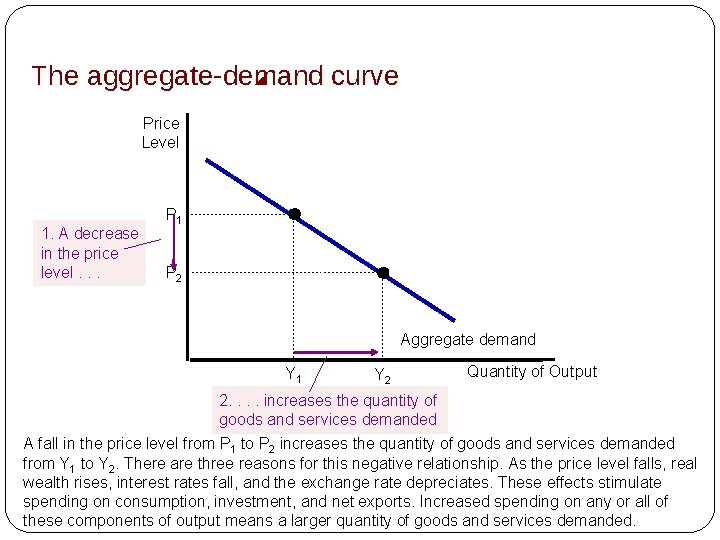The aggregate-demand curve Price Level 1. A decrease in the price level. . . P 1 P 2 Aggregate demand Y 1 Y 2 Quantity of Output 2. . increases the quantity of goods and services demanded A fall in the price level from P 1 to P 2 increases the quantity of goods and services demanded from Y 1 to Y 2. There are three reasons for this negative relationship. As the price level falls, real wealth rises, interest rates fall, and the exchange rate depreciates. These effects stimulate spending on consumption, investment, and net exports. Increased spending on any or all of these components of output means a larger quantity of goods and services demanded.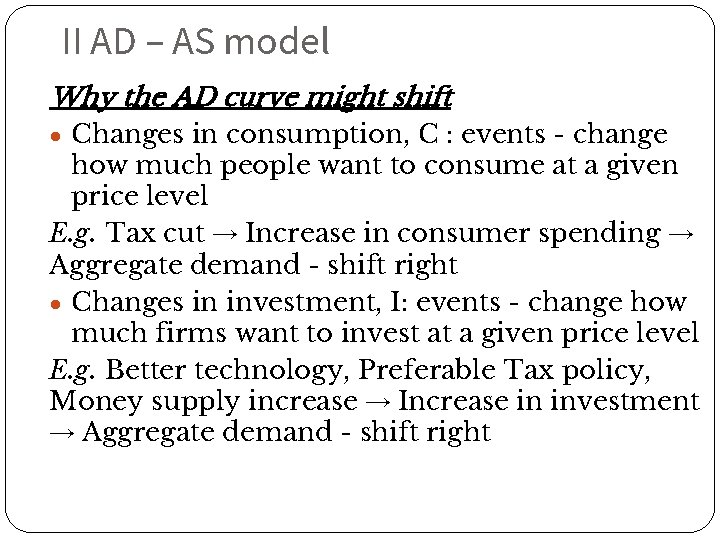II AD – AS model Why the AD curve might shift ● Changes in consumption, C : events - change how much people want to consume at a given price level E. g. Tax cut → Increase in consumer spending → Aggregate demand - shift right ● Changes in investment, I: events - change how much firms want to invest at a given price level E. g. Better technology, Preferable Tax policy, Money supply increase → Increase in investment → Aggregate demand - shift right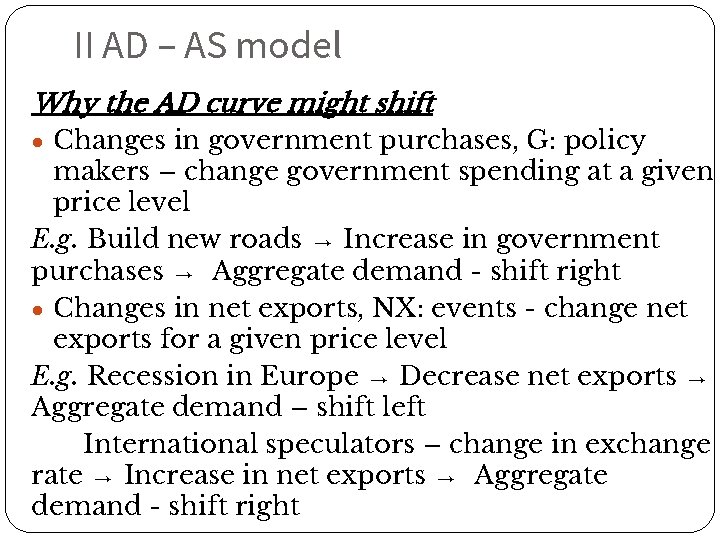II AD – AS model Why the AD curve might shift ● Changes in government purchases, G: policy makers – change government spending at a given price level E. g. Build new roads → Increase in government purchases → Aggregate demand - shift right ● Changes in net exports, NX: events - change net exports for a given price level E. g. Recession in Europe → Decrease net exports → Aggregate demand – shift left International speculators – change in exchange rate → Increase in net exports → Aggregate demand - shift rightThe aggregate-demand curve: summary (a) Why Does the Aggregate-Demand Curve Slope Downward? 1. The Wealth Effect: A lower price level increases real wealth, which stimulates spending on consumption. 2. The Interest-Rate Effect: A lower price level reduces the interest rate, which stimulates spending on investment. 3. The Exchange-Rate Effect: A lower price level causes the real exchange rate to depreciate, which stimulates spending on net exportsThe aggregate-demand curve: summary (b) Why Might the Aggregate-Demand Curve Shift? 1. Shifts Arising from Consumption: An event that makes consumers spend more at a given price level (a tax cut, a stock-market boom) shifts the aggregate-demand curve to the right. An event that makes consumers spend less at a given price level (a tax hike, a stock-market decline) shifts the aggregate-demand curve to the left. 2. Shifts Arising from Investment: An event that makes firms invest more at a. given price level (optimism about the future, a fall in interest rates due to an increase in the money supply) shifts the aggregate-demand curve to the right. An event that makes firms invest less at a given price level (pessimism about the future, a rise in interest rates due to a decrease in the money supply) shifts the aggregate-demand curve to the left. 3. Shifts Arising from Government Purchases: An increase in government purchases of goods and services (greater spending on defense or highway construction) shifts the aggregate-demand curve to the right. A decrease in government purchases on goods and services (a cutback in defense or highway spending) shifts the aggregate-demand curve to the left. 4. Shifts Arising from Net Exports: An event that raises spending on net exports at a given price level (a boom overseas, speculation that causes an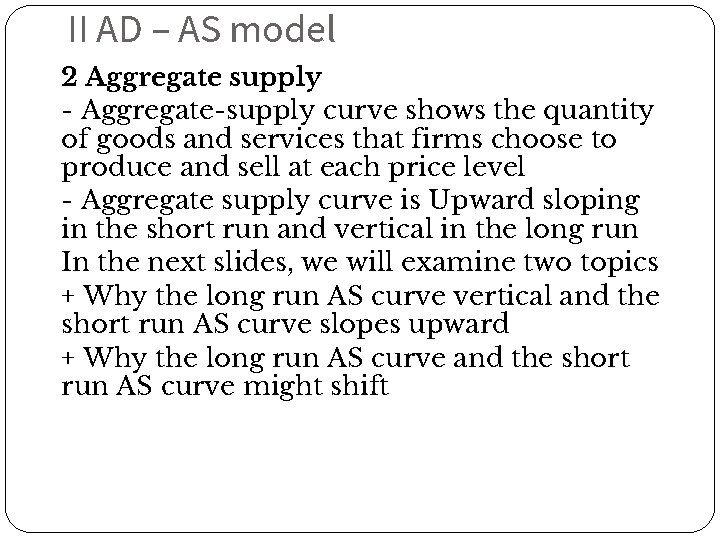II AD – AS model 2 Aggregate supply - Aggregate-supply curve shows the quantity of goods and services that firms choose to produce and sell at each price level - Aggregate supply curve is Upward sloping in the short run and vertical in the long run In the next slides, we will examine two topics + Why the long run AS curve vertical and the short run AS curve slopes upward + Why the long run AS curve and the short run AS curve might shift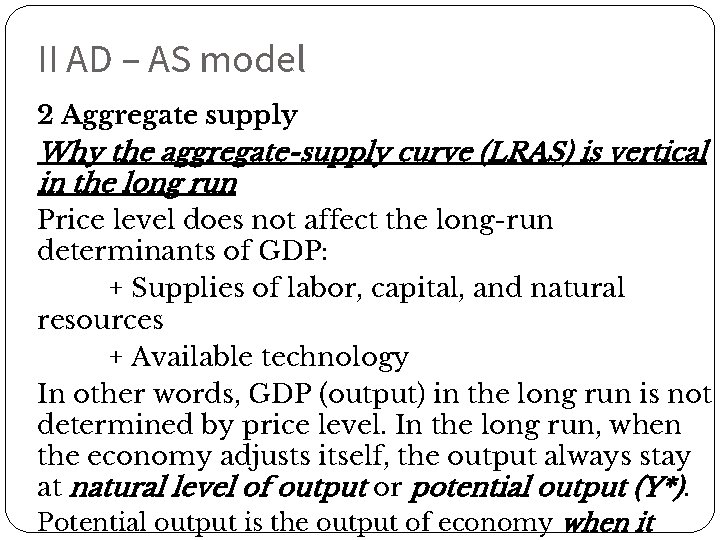II AD – AS model 2 Aggregate supply Why the aggregate-supply curve (LRAS) is vertical in the long run Price level does not affect the long-run determinants of GDP: + Supplies of labor, capital, and natural resources + Available technology In other words, GDP (output) in the long run is not determined by price level. In the long run, when the economy adjusts itself, the output always stay at natural level of output or potential output (Y*). Potential output is the output of economy when itThe long-run aggregate-supply curve Price Level Long-run aggregate supply P 1 1. A change in the price P 2 level. . . 2. . does not affect the quantity of goods and services supplied in the long run Natural level of output Quantity of Output In the long run, the quantity of output supplied depends on the economy’s quantities of labor, capital, and natural resources and on the technology for turning these inputs into output. Because the quantity supplied does not depend on the overall price level, the long-run aggregate-supply curve is vertical at the natural rate of output.II AD – AS model 2 Aggregate supply Why the LRAS curve might shift ● Changes in labor E. g. Quantity of labor – increases → Aggregate supply – shifts right Natural rate of unemployment – increases → Aggregate supply –shifts left ● Changes in capital E. g. Capital stock – decrease → Aggregate supply – shifts left ● Changes in natural resources E. g. New discovery of natural resource → Aggregate supply – shifts right Weather keeps fine → Aggregate supply – shifts right Availability of natural resources declines → Aggregate supply – shifts left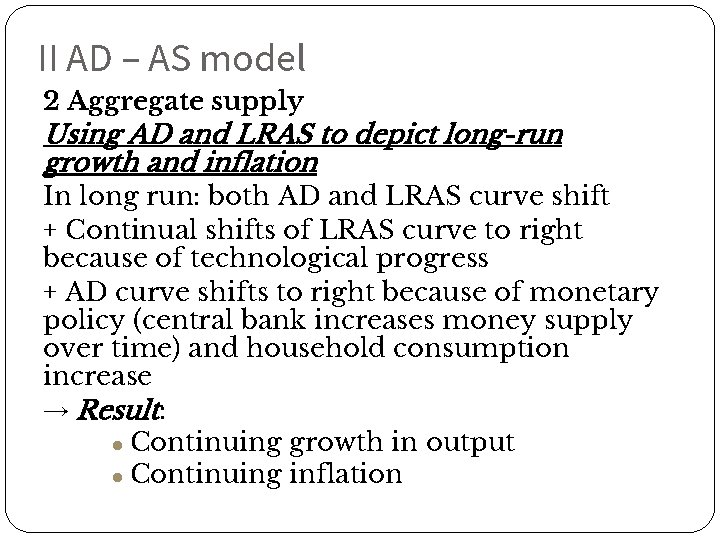II AD – AS model 2 Aggregate supply Using AD and LRAS to depict long-run growth and inflation In long run: both AD and LRAS curve shift + Continual shifts of LRAS curve to right because of technological progress + AD curve shifts to right because of monetary policy (central bank increases money supply over time) and household consumption increase → Result: ● Continuing growth in output ● Continuing inflationLong-run growth and inflation in the model of aggregate demand aggregate supply Price Level 2. . and growth in the money supply shifts aggregate demand. . . 1. In the long run, technological progress shifts long-run aggregate Long-run aggregate supply, supply… LRAS 1980 LRAS 1990 LRAS 2000 P 2000 3. . leading to growth in output. . . P 1990 P 1980 AD 2000 4. . and ongoing inflation AD 1980 Y 1990 AD 1990 Y 2000 Quantity of Output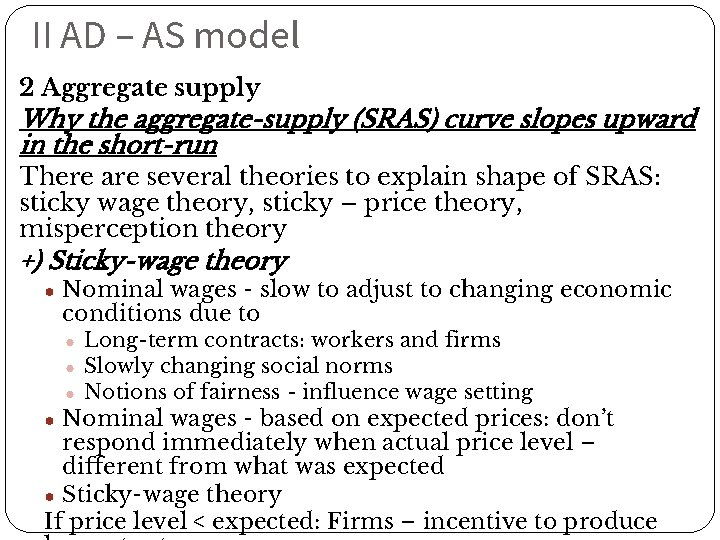II AD – AS model 2 Aggregate supply Why the aggregate-supply (SRAS) curve slopes upward in the short-run There are several theories to explain shape of SRAS: sticky wage theory, sticky – price theory, misperception theory +) Sticky-wage theory ● Nominal wages - slow to adjust to changing economic conditions due to ● Long-term contracts: workers and firms ● Slowly changing social norms ● Notions of fairness - influence wage setting ● Nominal wages - based on expected prices: don’t respond immediately when actual price level – different from what was expected ● Sticky-wage theory If price level < expected: Firms – incentive to produce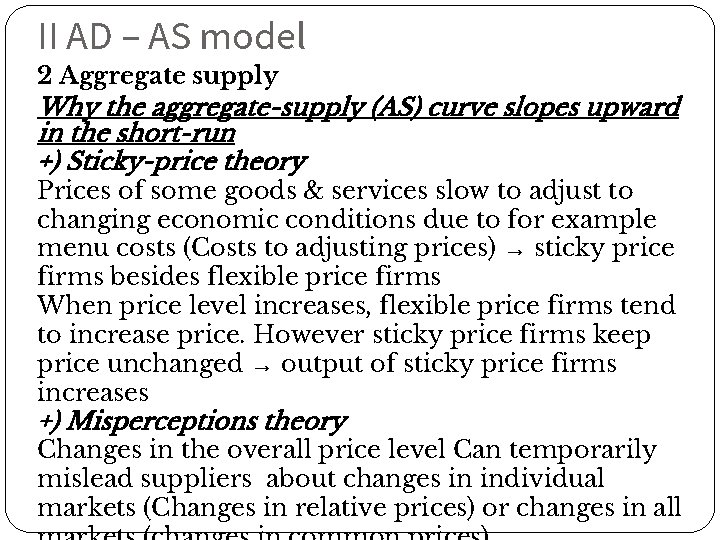II AD – AS model 2 Aggregate supply Why the aggregate-supply (AS) curve slopes upward in the short-run +) Sticky-price theory Prices of some goods & services slow to adjust to changing economic conditions due to for example menu costs (Costs to adjusting prices) → sticky price firms besides flexible price firms When price level increases, flexible price firms tend to increase price. However sticky price firms keep price unchanged → output of sticky price firms increases +) Misperceptions theory Changes in the overall price level Can temporarily mislead suppliers about changes in individual markets (Changes in relative prices) or changes in allII AD – AS model 2 Aggregate supply Using AD and SRAS to depict short run fluctuation of the economy a) Economy in normal b) Economy in recessionThe short-run aggregate-supply curve: summary (a) Why Does the Short-Run Aggregate-Supply Curve Slope Upward? 1. The Sticky-Wage Theory: An unexpectedly low price level raises the real wage, which causes firms to hire fewer workers and produce a smaller quantity of goods and services. 2. The Sticky-Price Theory: An unexpectedly low price level leaves some firms with higherthan desired prices, which depresses their sales and leads them to cut back production. 3. The Misperceptions Theory: An unexpectedly low price level leads some suppliers to think their relative prices have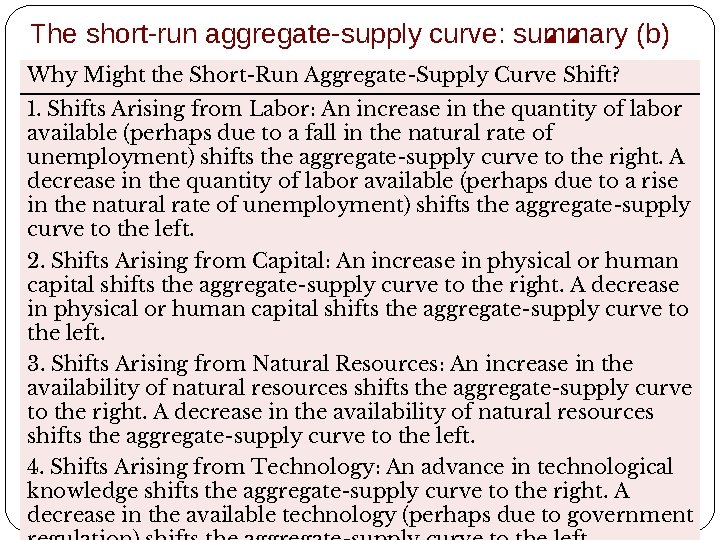The short-run aggregate-supply curve: summary (b) Why Might the Short-Run Aggregate-Supply Curve Shift? 1. Shifts Arising from Labor: An increase in the quantity of labor available (perhaps due to a fall in the natural rate of unemployment) shifts the aggregate-supply curve to the right. A decrease in the quantity of labor available (perhaps due to a rise in the natural rate of unemployment) shifts the aggregate-supply curve to the left. 2. Shifts Arising from Capital: An increase in physical or human capital shifts the aggregate-supply curve to the right. A decrease in physical or human capital shifts the aggregate-supply curve to the left. 3. Shifts Arising from Natural Resources: An increase in the availability of natural resources shifts the aggregate-supply curve to the right. A decrease in the availability of natural resources shifts the aggregate-supply curve to the left. 4. Shifts Arising from Technology: An advance in technological knowledge shifts the aggregate-supply curve to the right. A decrease in the available technology (perhaps due to governmentIII Explain behaviors of the economy via AD – AS model Two causes of economic fluctuations: shift of the AD curve and shift of the SRAS curve We begin short run examination with ● Assumption: Economy begins in long-run equilibrium ● Long-run equilibrium: Intersection of AD and LRAS curve (Output - natural rate ; Actual price level) and Intersection of AD and short-run AS curve (Expected price level = Actual price level)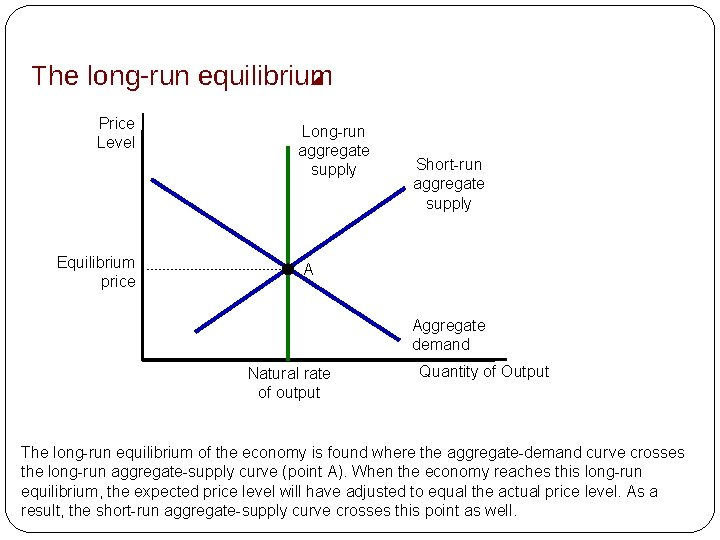The long-run equilibrium Price Level Equilibrium price Long-run aggregate supply Short-run aggregate supply A Aggregate demand Natural rate of output Quantity of Output The long-run equilibrium of the economy is found where the aggregate-demand curve crosses the long-run aggregate-supply curve (point A). When the economy reaches this long-run equilibrium, the expected price level will have adjusted to equal the actual price level. As a result, the short-run aggregate-supply curve crosses this point as well.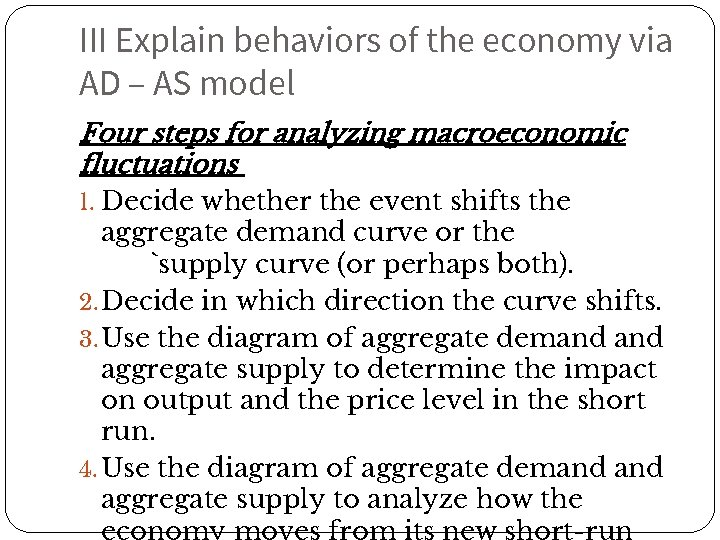III Explain behaviors of the economy via AD – AS model Four steps for analyzing macroeconomic fluctuations 1. Decide whether the event shifts the aggregate demand curve or the `supply curve (or perhaps both). 2. Decide in which direction the curve shifts. 3. Use the diagram of aggregate demand aggregate supply to determine the impact on output and the price level in the short run. 4. Use the diagram of aggregate demand aggregate supply to analyze how the economy moves from its new short-runIII Explain behaviors of the economy via AD – AS model 1 The effects of a shift in aggregate demand : expansionary demand shock and contractionary demand shock Contractionary demand shock Factor: Wave of pessimism affects aggregate demand → Aggregate demand – shifts left ● Short-run: Output falls & Price level falls ● Long-run: Short-run aggregate supply curve – shifts right → Output – natural rate and Price level – fallsA contraction in aggregate demand Price Level P 1 P 2 Long-run aggregate supply A B C P 3 Short-run aggregate supply, AS 1 AS 2 3. . but over time, the short-run aggregate-supply curve shifts. . . 4. . and output returns to its natural rate. 1. A decrease in aggregate demand. . . AD 2 Y 2 Aggregate demand, AD 1 Y 1 Quantity of Output 2. . causes output to fall in the short run. . . A fall in aggregate demand is represented with a leftward shift in the aggregate-demand curve from AD 1 to AD 2. In the short run, the economy moves from point A to point B. Output falls from Y 1 to Y 2, and the price level falls from P 1 to P 2. Over time, as the expected price level adjusts, the short-run aggregate-supply curve shifts to the right from AS 1 to AS 2, and the economy reaches point C, where the new aggregate-demand curve crosses the long-run aggregatesupply curve. In the long run, the price level falls to P 3, and output returns to its natural rate, Y 1.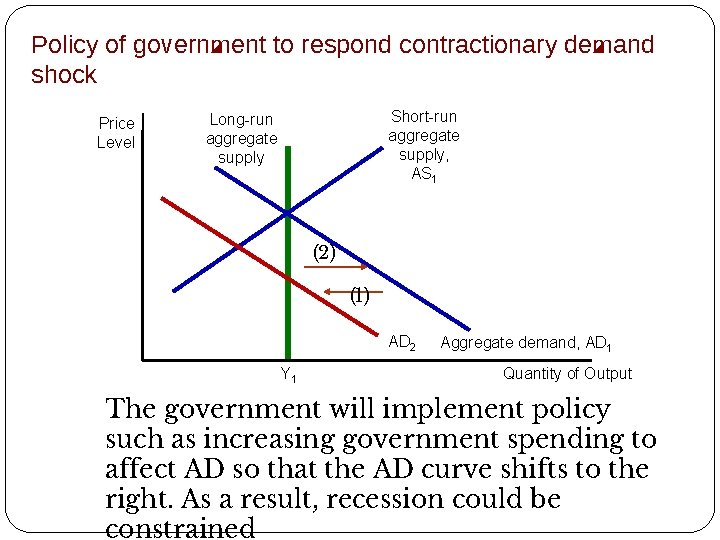Policy of government to respond contractionary demand shock Price Level Short-run aggregate supply, AS 1 Long-run aggregate supply (2) (1) AD 2 Y 1 Aggregate demand, AD 1 Quantity of Output The government will implement policy such as increasing government spending to affect AD so that the AD curve shifts to the right. As a result, recession could be constrainedIII Explain behaviors of the economy via AD – AS model 1 The effects of a shift in aggregate demand : expansionary demand shock and contractionary demand shock Expansionary demand shock Factor: Decrease of interest rate affects aggregate demand → Aggregate demand – shifts left ● Short-run: Output rises & Price level increases ● Long-run: Short-run aggregate supply curve – shifts left → Output – natural rate and Price level – rises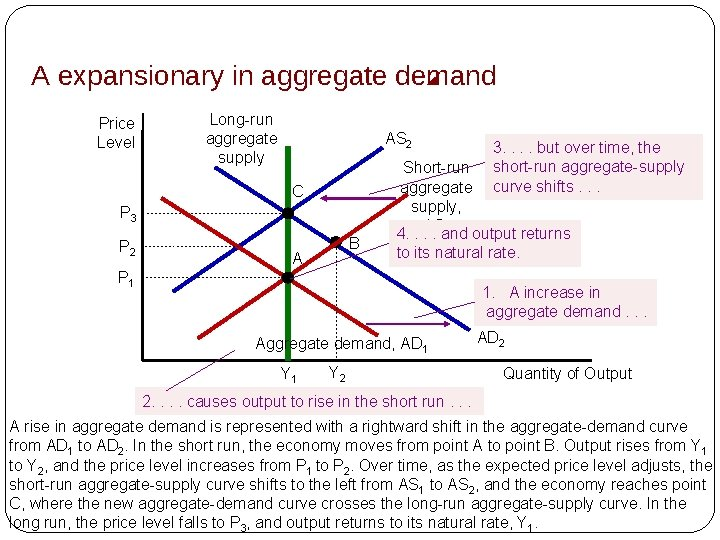A expansionary in aggregate demand Price Level Long-run aggregate supply AS 2 C P 3 P 2 B A 3. . but over time, the short-run aggregate-supply curve shifts. . . Short-run aggregate supply, AS 1 4. . and output returns to its natural rate. P 1 1. A increase in aggregate demand. . . Aggregate demand, AD 1 Y 2 AD 2 Quantity of Output 2. . causes output to rise in the short run. . . A rise in aggregate demand is represented with a rightward shift in the aggregate-demand curve from AD 1 to AD 2. In the short run, the economy moves from point A to point B. Output rises from Y 1 to Y 2, and the price level increases from P 1 to P 2. Over time, as the expected price level adjusts, the short-run aggregate-supply curve shifts to the left from AS 1 to AS 2, and the economy reaches point C, where the new aggregate-demand curve crosses the long-run aggregate-supply curve. In the long run, the price level falls to P 3, and output returns to its natural rate, Y 1.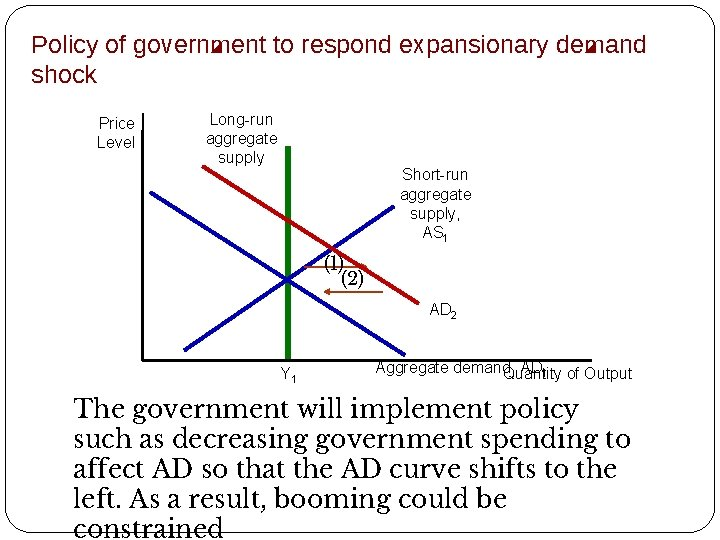Policy of government to respond expansionary demand shock Price Level Long-run aggregate supply Short-run aggregate supply, AS 1 (1) (2) AD 2 Y 1 Aggregate demand, AD 1 of Output Quantity The government will implement policy such as decreasing government spending to affect AD so that the AD curve shifts to the left. As a result, booming could be constrainedIII Explain behaviors of the economy via AD – AS model 1 The effects of a shift in aggregate demand : expansionary demand shock and contractionary demand shock Summary AD curve Output shift to Price level (inflation) Policy of government Contracti on Left Decline (short run) Unchanged (long run) Fall (short run) Fall further (long run) Shift AD to the right Expansio n Right Rise (short run) Unchanged (long run) Increase (short run) Increase further (long run) Shift AD to the left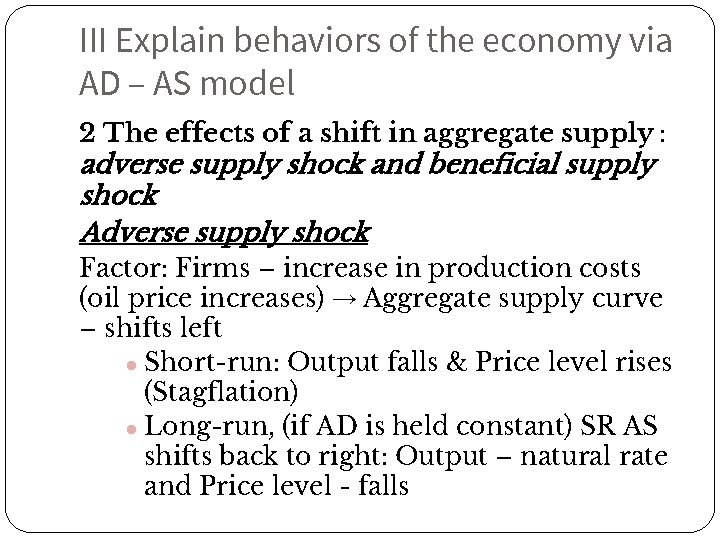III Explain behaviors of the economy via AD – AS model 2 The effects of a shift in aggregate supply : adverse supply shock and beneficial supply shock Adverse supply shock Factor: Firms – increase in production costs (oil price increases) → Aggregate supply curve – shifts left ● Short-run: Output falls & Price level rises (Stagflation) ● Long-run, (if AD is held constant) SR AS shifts back to right: Output – natural rate and Price level - fallsAn adverse shift in aggregate supply Price Level Long-run aggregate supply AS 2 1. An adverse shift in the short-run aggregate-supply curve. . . Short-run aggregate supply, AS 1 B P 2 3. . and the price P 1 level to rise A 4. Long run, AS shifts back Aggregate demand Y 2 Y 1 Quantity of Output 2. . causes output to fall. . . When some event increases firms’ costs, the short-run aggregate-supply curve shifts to the left from AS 1 to AS 2. The economy moves from point A to point B. The result is stagflation: Output falls from Y 1 to Y 2, and the price level rises from P 1 to P 2.Policy of government to respond adverse supply shock Price Level Long-run aggregate supply AS 2 Short-run aggregate supply, AS 1 Econo my Gov( 2) Gov( 1) Y 1 Aggregate demand Quantity of Output Government has 2 choices: + gov(1) decrease AD → shift AD to the left → keep P unchanged but Y declines further + gov(2) increase AD → shift AD to the right → keep Y unchanged but Y rises further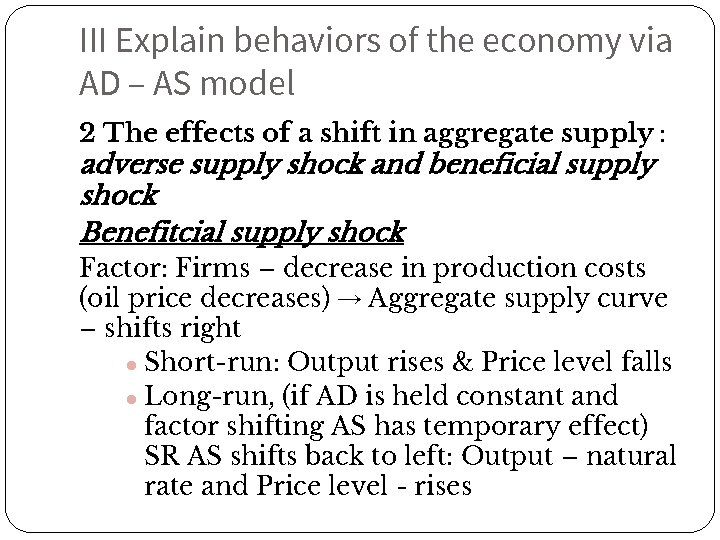III Explain behaviors of the economy via AD – AS model 2 The effects of a shift in aggregate supply : adverse supply shock and beneficial supply shock Benefitcial supply shock Factor: Firms – decrease in production costs (oil price decreases) → Aggregate supply curve – shifts right ● Short-run: Output rises & Price level falls ● Long-run, (if AD is held constant and factor shifting AS has temporary effect) SR AS shifts back to left: Output – natural rate and Price level - rises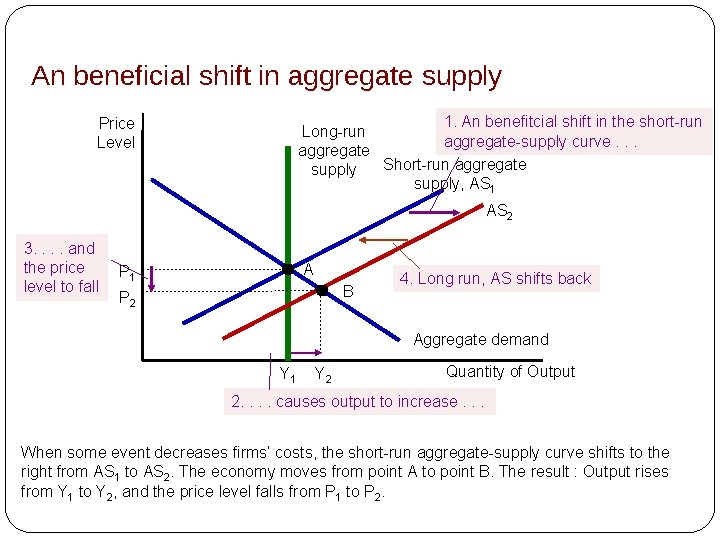An beneficial shift in aggregate supply 1. An benefitcial shift in the short-run Long-run aggregate-supply curve. . . aggregate Short-run aggregate supply, AS 1 Price Level AS 2 3. . and the price level to fall A P 1 B P 2 4. Long run, AS shifts back Aggregate demand Y 1 Y 2 Quantity of Output 2. . causes output to increase. . . When some event decreases firms’ costs, the short-run aggregate-supply curve shifts to the right from AS 1 to AS 2. The economy moves from point A to point B. The result : Output rises from Y 1 to Y 2, and the price level falls from P 1 to P 2.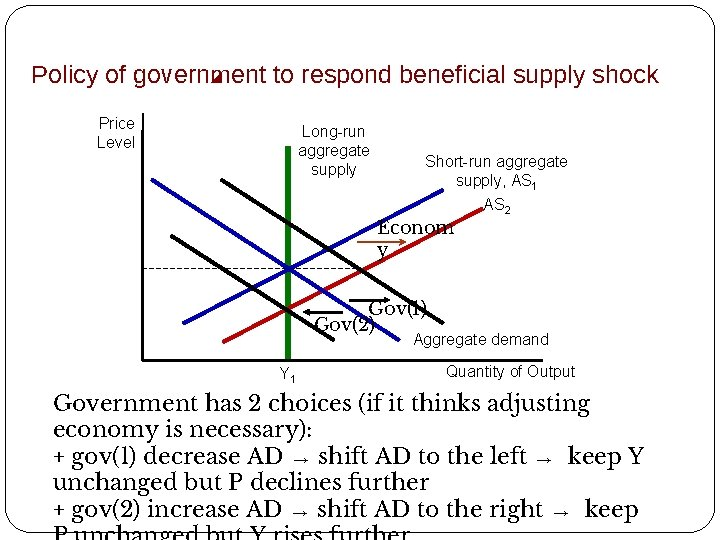Policy of government to respond beneficial supply shock Price Level Long-run aggregate supply Short-run aggregate supply, AS 1 Econom y AS 2 Gov(1) Gov(2) Aggregate demand Y 1 Quantity of Output Government has 2 choices (if it thinks adjusting economy is necessary): + gov(1) decrease AD → shift AD to the left → keep Y unchanged but P declines further + gov(2) increase AD → shift AD to the right → keep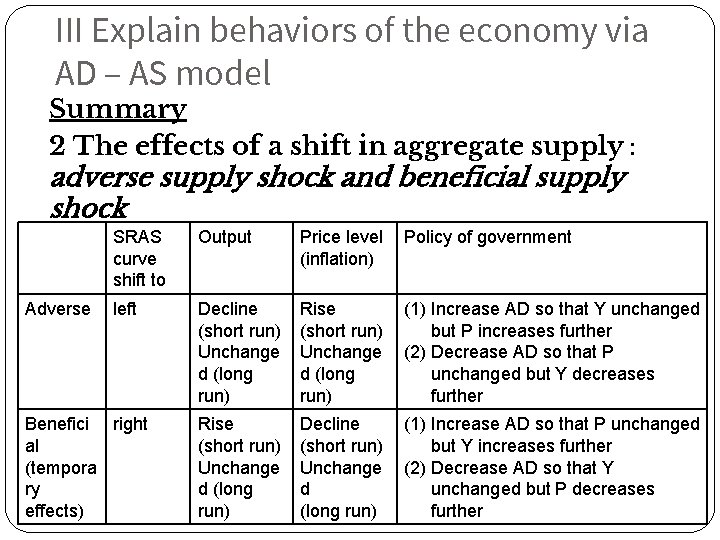III Explain behaviors of the economy via AD – AS model Summary 2 The effects of a shift in aggregate supply : adverse supply shock and beneficial supply shock Adverse SRAS curve shift to Output Price level (inflation) Policy of government left Decline (short run) Unchange d (long run) Rise (short run) Unchange d (long run) (1) Increase AD so that Y unchanged but P increases further (2) Decrease AD so that P unchanged but Y decreases further Rise (short run) Unchange d (long run) Decline (short run) Unchange d (long run) (1) Increase AD so that P unchanged but Y increases further (2) Decrease AD so that Y unchanged but P decreases further Benefici right al (tempora ry effects)Appendix 1 Closer look to supply shock 1) Adverse supply shock Price Level Long-run aggregate supply AS 2 AS 3 Short-run aggregate supply, AS 1 B A Aggregate demand Y 2 Y 1 Quantity of Output In the long run, the economy will not come back point A, initial long run equilibrium. Because, adverse supply shock potentially harms natural level of output then LRAS shift to the left. Eventually, in long run, the economy will move to the new equilibrium at point B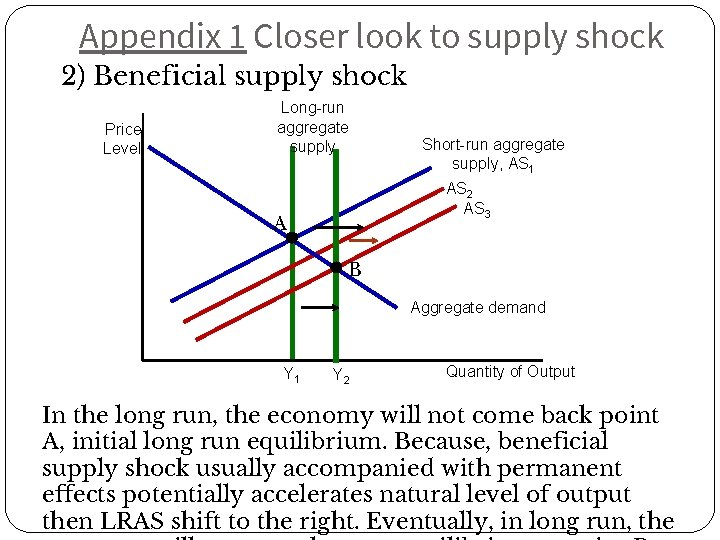Appendix 1 Closer look to supply shock 2) Beneficial supply shock Price Level Long-run aggregate supply Short-run aggregate supply, AS 1 AS 2 AS 3 A B Aggregate demand Y 1 Y 2 Quantity of Output In the long run, the economy will not come back point A, initial long run equilibrium. Because, beneficial supply shock usually accompanied with permanent effects potentially accelerates natural level of output then LRAS shift to the right. Eventually, in long run, theAppendix 2 Using vertical axis of inflation rate in AD – AS model Mathematical transformations formula of AS curve (follows Phillips curve equation) and AD curve (follow dynamic AD equation under Mundell – Fleming model), we both arrive at conclusion that: • In terms of aggregate supply, inflation rate and output have positive relationship • In terms oflnflation aggregate demand, inflation rate and output Short-run aggregate have negative relationship supply ∏e Aggregate demand Y* Quantity of OutputKey concepts • Aggregate demand • Aggregate supply • Wealth effect • Interest-rate effect • Exchange-rate effect • Sticky wage theory • Sticky price theory • Misperception theory • Potential output • Expansionary/Contractionary demand shock • Beneficial/Adverse supply shock